## Circle Theorems

Theorems

This section explains circle theorem, including tangents, sectors, angles and proofs.

The video below highlights the rules you need to remember to work out circle theorems.Isosceles Triangle

Two Radii and a chord make an isosceles triangle.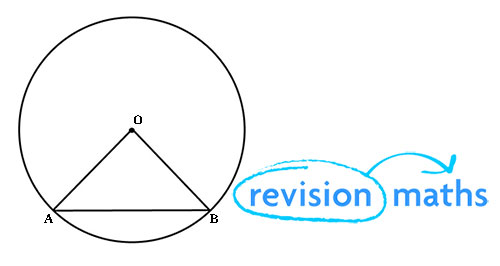Perpendicular Chord Bisection

The perpendicular from the centre of a circle to a chord will always bisect the chord (split it into two equal lengths).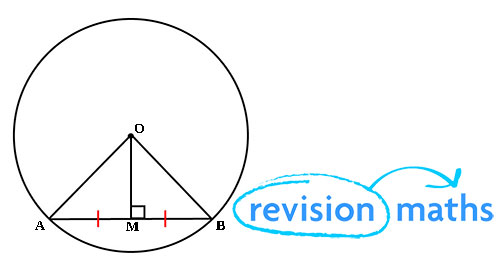Angles Subtended on the Same Arc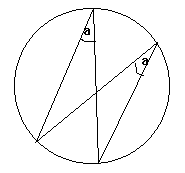Angles formed from two points on the circumference are equal to other angles, in the same arc, formed from those two points.

Angle in a Semi-Circle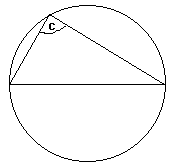Angles formed by drawing lines from the ends of the diameter of a circle to its circumference form a right angle. So c is a right angle.

Proof

We can split the triangle in two by drawing a line from the centre of the circle to the point on the circumference our triangle touches.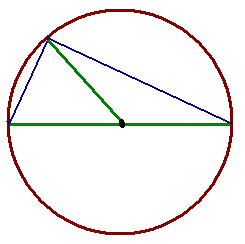We know that each of the lines which is a radius of the circle (the green lines) are the same length. Therefore each of the two triangles is isosceles and has a pair of equal angles.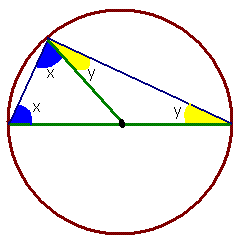But all of these angles together must add up to 180°, since they are the angles of the original big triangle.

Therefore x + y + x + y = 180, in other words 2(x + y) = 180.
and so x + y = 90. But x + y is the size of the angle we wanted to find.

Tangents

A tangent to a circle is a straight line which touches the circle at only one point (so it does not cross the circle- it just touches it).

A tangent to a circle forms a right angle with the circle's radius, at the point of contact of the tangent.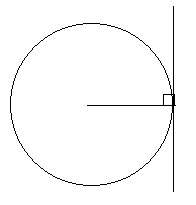Also, if two tangents are drawn on a circle and they cross, the lengths of the two tangents (from the point where they touch the circle to the point where they cross) will be the same.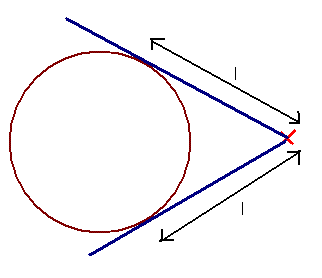Angle at the Centre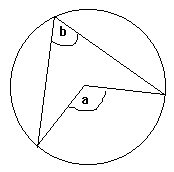The angle formed at the centre of the circle by lines originating from two points on the circle's circumference is double the angle formed on the circumference of the circle by lines originating from the same points. i.e. a = 2b.

Proof

You might have to be able to prove this fact: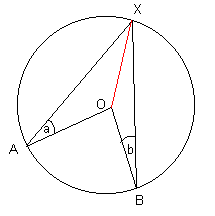OA = OX since both of these are equal to the radius of the circle. The triangle AOX is therefore isosceles and so ∠OXA = a
Similarly, ∠OXB = b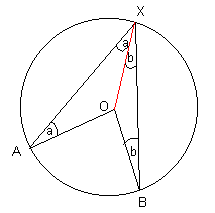Since the angles in a triangle add up to 180, we know that ∠XOA = 180 - 2a
Similarly, ∠BOX = 180 - 2b
Since the angles around a point add up to 360, we have that ∠AOB = 360 - ∠XOA - ∠BOX
= 360 - (180 - 2a) - (180 - 2b)
= 2a + 2b = 2(a + b) = 2 ∠AXB

Alternate Segment Theorem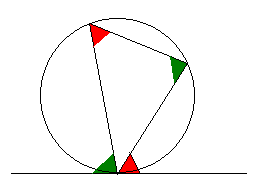This diagram shows the alternate segment theorem. In short, the red angles are equal to each other and the green angles are equal to each other.

Proof

You may have to be able to prove the alternate segment theorem: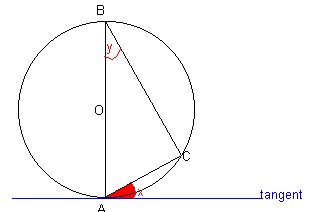We use facts about related angles

A tangent makes an angle of 90 degrees with the radius of a circle, so we know that ∠OAC + x = 90.
The angle in a semi-circle is 90, so ∠BCA = 90.
The angles in a triangle add up to 180, so ∠BCA + ∠OAC + y = 180
Therefore 90 + ∠OAC + y = 180 and so ∠OAC + y = 90
But OAC + x = 90, so ∠OAC + x = ∠OAC + y
Hence x = y

A cyclic quadrilateral is a four-sided figure in a circle, with each vertex (corner) of the quadrilateral touching the circumference of the circle. The opposite angles of such a quadrilateral add up to 180 degrees.

Area of Sector and Arc Length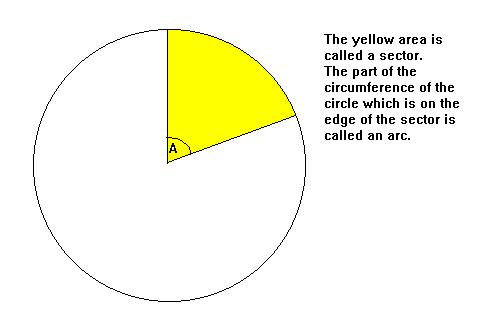If the radius of the circle is r,
Area of sector = πr2 × A/360
Arc length = 2πr × A/360

In other words, area of sector = area of circle × A/360
arc length = circumference of circle × A/360